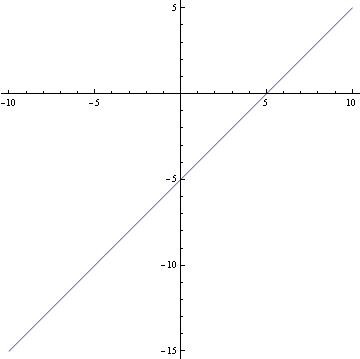Find The Slope,if Exists:y=-7x+9;graph The Linear Equation: X=y+5?

1. The slope of a line whose equation is in slope-intercept form is given by the coefficient of x. The slope is -7.

2. The equation can be graphed as is, or it can be put into standard form or slope-intercept form to aid in graphing. Standard form:

x - y = 5. Slope-intercept form:

y = x - 5. Intercept form:

x/5 + y/(-5) = 1.thanked the writer.